Question

Test the hypothesis using the​ P-value approach. Be sure to verify the requirements of the test. H0​: P =0.3 versus H 1: P >0.3 n =200 x =70 α =0.01

What is the P value? Round to the nearest three decimal places.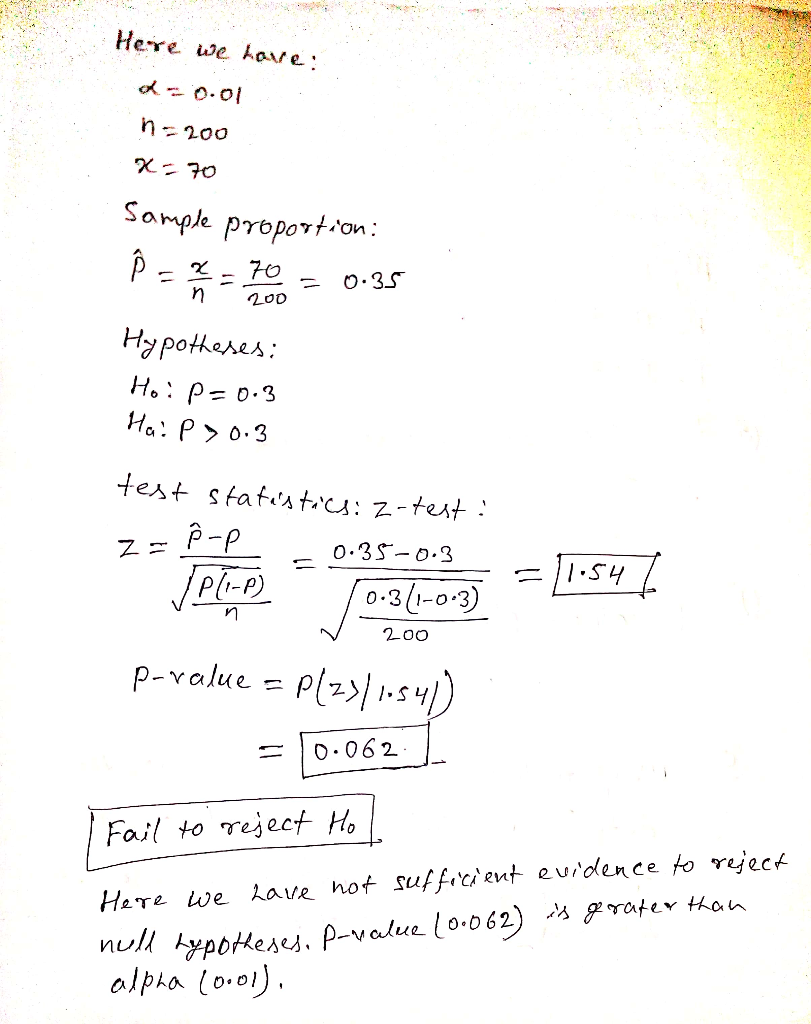#### Earn Coins

Coins can be redeemed for fabulous gifts.

Similar Homework Help Questions
• ### Test the hypothesis using the​ P-value approach. Be sure to verify the requirements of the test....

Test the hypothesis using the​ P-value approach. Be sure to verify the requirements of the test. H0​: p=0.6 versus H1​: p>0.6 n=250; x=160​, a=0.01 Is np 0 ( 1−p0) ≥​10? Yes No Use technology to find the​ P-value. ​P- value=________ ​(Round to three decimal places as​ needed.) __________the null​ hypothesis, because the​ P-value is __________ than α.

• ### Test the hypothesis using the P-value approach. Be sure to verify the requirements of the test....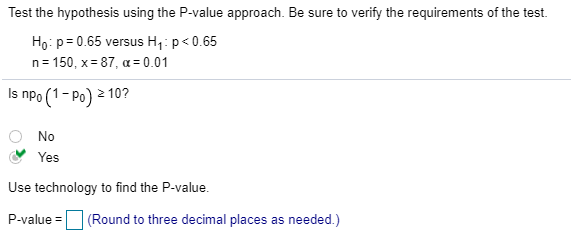Test the hypothesis using the P-value approach. Be sure to verify the requirements of the test. Ho: p = 0.65 versus H : p<0.65 n= 150, x= 87, a = 0.01 Is npo (1-po) 2 10? Ο Νο Yes Use technology to find the P-value. P-value = (Round to three decimal places as needed.)

• ### Test the hypothesis using the P-value approach Be sure to verify the requirements of the test...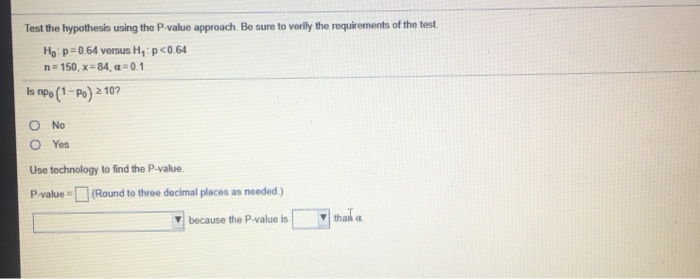Test the hypothesis using the P-value approach Be sure to verify the requirements of the test Ho p=0.64 versus H, p<0.64 n=150, x=84 c = 0.1 Is npo (1-Po) 210? O No O Yes Use technology to find the P-value P value - (Round to three decimal places as needed) because the P value is that a

• ### Test the hypothesis using the P-value approach. Be sure to verify the requirements of the test...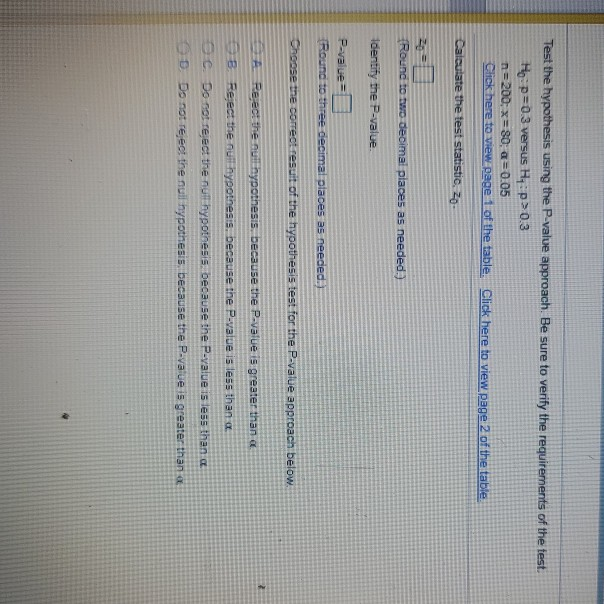Test the hypothesis using the P-value approach. Be sure to verify the requirements of the test Ho p=0.3 versus H:p>0.3 n=200: x=80; a=0.05 Click here to view page 1 of the table. Click here to view page 2 of the table Calculate the test statistic. zo- {Round to two decimal places as needed.) Identify the P-value P-value Round to three decimal places as needed. Choose the correct result of the hypothesis test for the P-value approach below. al Reject the...

• ### Test the hypothesis using the P-value approach. Be sure to verify the requirements of the test....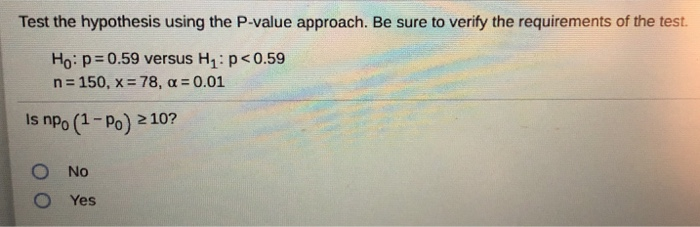Test the hypothesis using the P-value approach. Be sure to verify the requirements of the test. Ho: p=0.59 versus H : p<0.59 n = 150, x= 78, a = 0.01 Is npo (1 - Po) 210? Ο O Νο Yes

• ### 10.2.7-T Test the hypothesis using the P-value approach. Be sure to verify the requirements of the...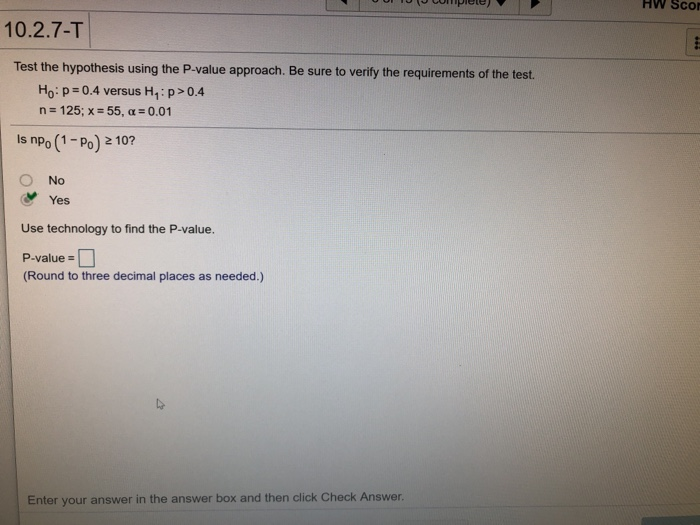10.2.7-T Test the hypothesis using the P-value approach. Be sure to verify the requirements of the test. Ho: p-o4 versus H1 : p-o4 n 125; x-55, a 0.01 Is npo (1 -Po) 2 10? O No Yes Use technology to find the P-value. P-value - (Round to three decimal places as needed.) Enter your answer in the answer box and then click Check Answer.

• ### Test the hypothesis using the P-value approach. Be sure to verify the requirements of the test....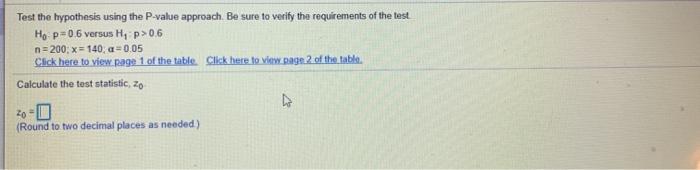Test the hypothesis using the P-value approach. Be sure to verify the requirements of the test. Ho: p=0.6 versus Hy p>0.6 n = 200; x = 140; a = 0.05 Click here to view page 1 of the table. Click here to view.page 2 of the table. Calculate the test statistic, 20- (Round to two decimal places as needed)

• ### on Test the hypothesis using the P-value approach. Be sure to verify the requirements of the...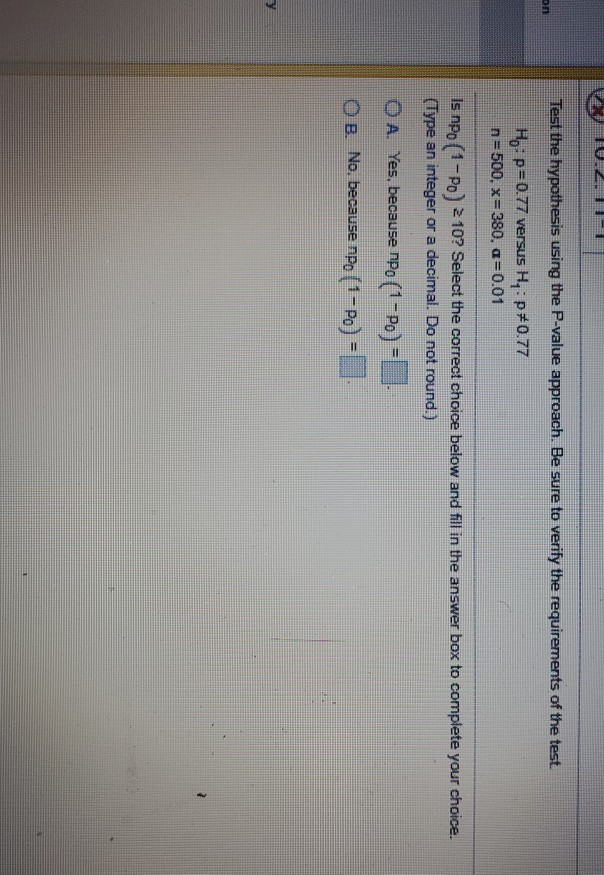on Test the hypothesis using the P-value approach. Be sure to verify the requirements of the test. Ho: p=0.77 versus H, :p*0.77 n=500, x=380. a=0.01 Is npo (1-P) 2 10? Select the correct choice below and fill in the answer box to complete your choice. (Type an integer or a decimal. Do not round.) OA. Yes, because npo (1-Po) - OB. No, because npo (1-P) =

• ### help please Test the hypothesis using the P-value approach. Be sure to verify the requirements of...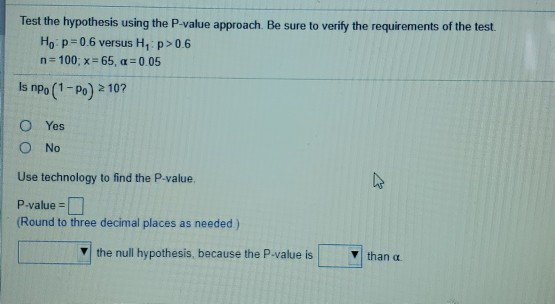help please Test the hypothesis using the P-value approach. Be sure to verify the requirements of the test. Ho: p=0.6 versus H, p>0.6 n=100; x = 65, a = 0.05 Is npo (1-P) 2107 0 Yes O No Use technology to find the P-value, P-value=0 (Round to three decimal places as needed.) the null hypothesis, because the P-value is than a

• ### Test the hypothesis using the P-value approach. Be sure to verify the requirements of the test....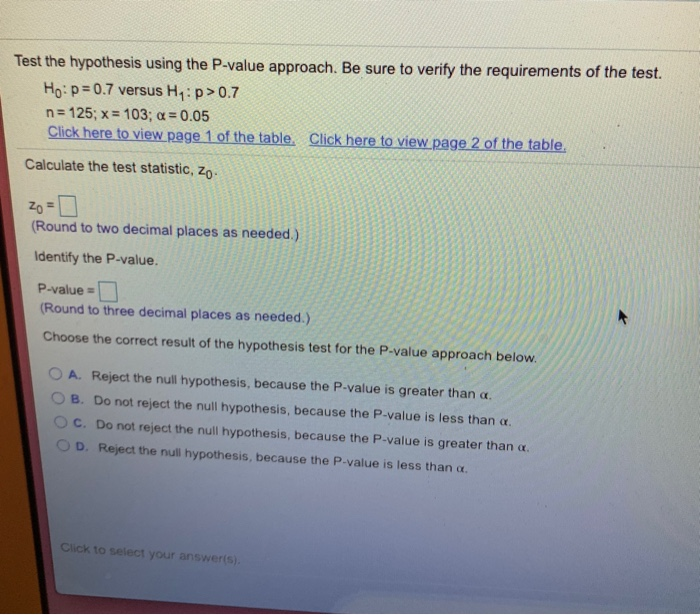Test the hypothesis using the P-value approach. Be sure to verify the requirements of the test. Ho: p=0.7 versus H,:>0.7 n = 125; x = 103; a = 0.05 Click here to view page 1 of the table. Click here to view page 2 of the table. Calculate the test statistic, Zo Zo - (Round to two decimal places as needed.) Identify the P-value. P-value = (Round to three decimal places as needed.) Choose the correct result of the hypothesis...